These notes are meant to serve as an introduction to topological data analysis (TDA).

I’m interested in its applicability to neuroscience, AI and (deep) learning. I’m convinced that it’s the right level of generality for certain problems.

## What is topological data analysis?

an approach to the analysis of datasets using techniques from topology – Wikipedia

A more detailed answer might be: TDA involves ‘fitting’ a topological space to data, then perhaps computing topological invariants of that space.

TDA is related to two familiar problems: clustering and manifold learning. In some sense, TDA is a generalization of both problems.

## What is topology?

• Topology is qualitative geometry

• Topology is the study of shape and space

• Topology is the study of badly drawn figures – Howard Eves

Topology is one of the main branches of mathematics. It is as foundational as algebra and analysis. Like geometry, topology is the study of space. Whereas geometry uses a quantitative notion of distance between two points (a metric), topology uses a qualitative notion of distance (via the language of open sets).

All (geo)metric spaces are topological spaces, but not all topological spaces are metric spaces.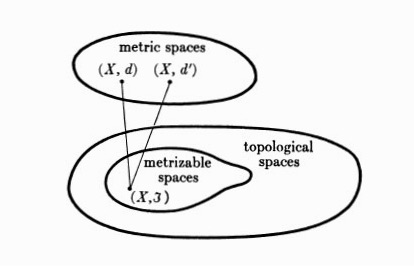One way to study spaces is to study the maps between spaces. Hence, topology is just as much about the study of spaces as it is about the continuous functions between them, just as linear algebra is equal parts the study of vector spaces and the linear functions between them.

A crash course on topology,

## Simplicial complexes

We can view many data analysis problems as ‘fitting a space to data’. E.g. both PCA and linear regression involve fitting a linear subspace to data; the space perspective complements the statistical (least-squares) and algebraic (SVD, pseudoinverse) perspectives of these techniques.

How do we generalize these linear techniques? A stock answer: manifold learning. But, manifolds are restrictive objects. A consequence of being locally Euclidean is that they cannot contain singularities and must have the same dimension everywhere. A mathematician’s motivation for working with manifolds is that one can do calculus on them. But if this is not the goal, then there may be little reason to assume a restrictive type of space in a data analysis context. Indeed, the singularities of a space are often the interesting points of study (e.g. bifurcation points), and one wants tools to capture these.

So, topological spaces are a natural thing to turn to whenever one wants to ‘fit a space to data’. Yet topological spaces, without restriction, are too general (there are many pathological spaces). Fortunatley, many classes of discrete / combinatorial / finite topological spaces avoid these pathologies, while still being far more general (and potentially far more useful) than manifolds. A good example: simplicial complexes, which can be viewed as a convenient middle ground of specificity and generality in modeling spaces.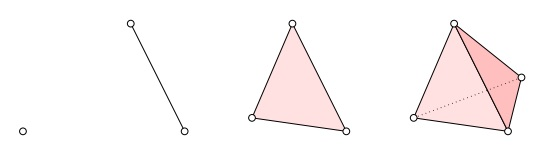Simplices: discrete building blocks for topological spaces.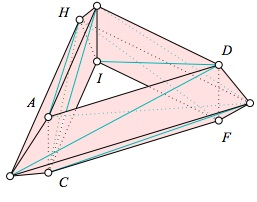A torus as a simplicial complex. Images from here.

Simplicial complexes lie somewhere between graphs and hypergraphs. All graphs are special kinds of simplicial complexes, and all simplicial complexes are special kinds of hypergraphs. “Most” topological spaces of interest can be discretized (triangulated) and represented as a simplicial complex.

## Persistent homology

The workhorse technique of TDA is persistent homology. Instead of outlining the technique here, the gist of it can be efficiently surmised from,

Persistent homology “generalizes clustering” in two ways: first, that it includes higher-order homological features in addition to the 0th order feature (i.e. the clusters); second, that it includes a persitence parameter that tells us what homological features exist at which scales. One only has to look to the ubiquity of clustering to see that persistent homology is a sensible thing to do.

## Mapper

An especially underused TDA technique is “mapper”. I found this particularly useful for visualization, and I wonder why it isn’t as widely applied as, say, t-SNE. Mapper fits a simplicial complex to data (often, just a graph), but in a very flexible way. The goal of mapper is either data visualization or clustering.

The key insight offered by this technique is that many interesting “clusters” in real data are not clusters in the classical sense (as disconnected components), but are the branches of some single connected component. Think about the three “clusters” in the shape Y. As simple as this sounds, this insight has been driving real progress in cancer genomics (where the “clusters” are rarely true clusters), and I suspect this method (or some reinvention of it) will find its ways into more fields in due time.

The original paper describing the technique is here, but a perhaps better reference is the Scientific Reports paper:

The result of mapper on data from monkey motor cortex during reaching movements: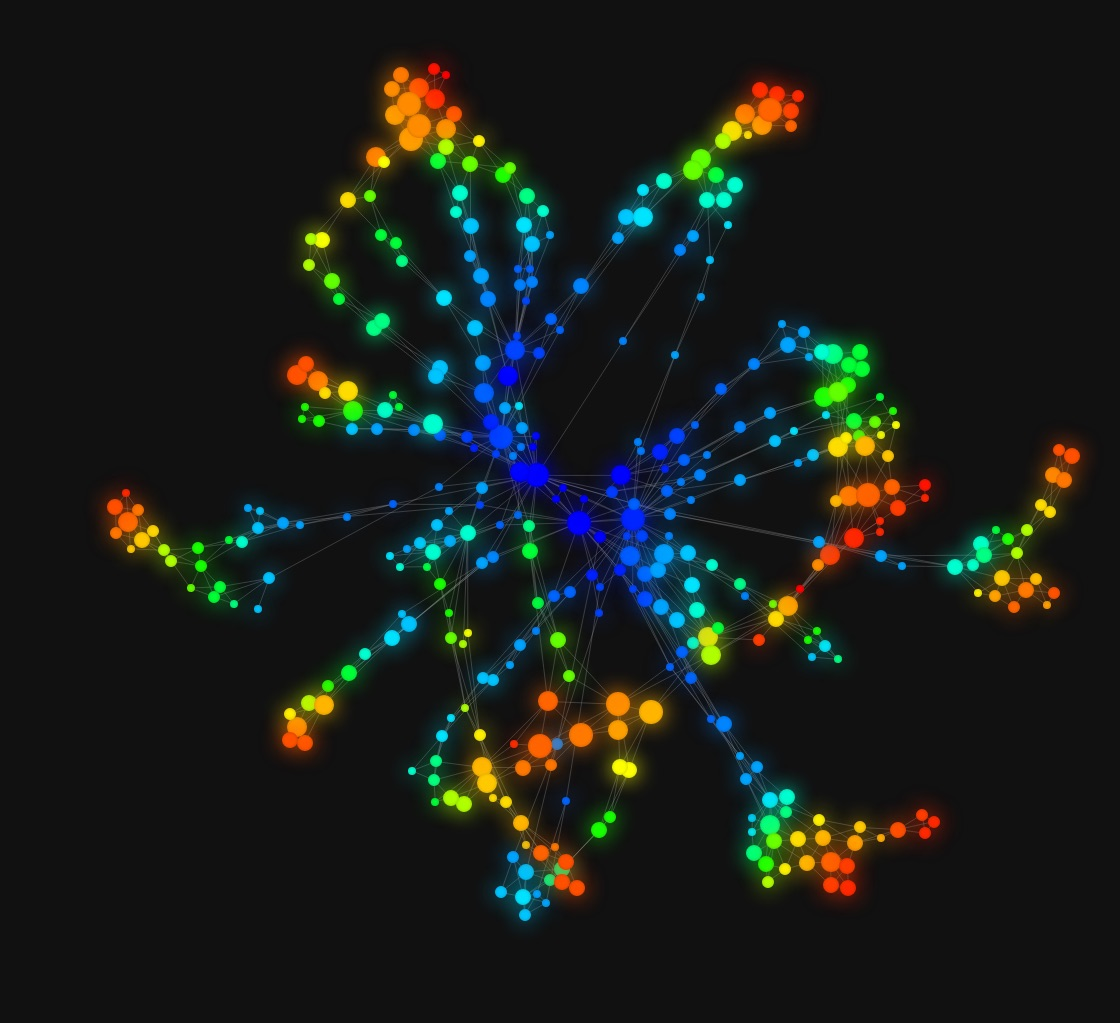Animated mapper visuals: one, two, three, four.

The technique could benefit from more open source treatment. Myself and others have had too much difficulty using Python mapper. The above visuals were created using KeplerMapper, which conveniently interfaces with scikit-learn’s clustering API. Better software exists, but is not free.

## TDA in neuroscience

There is a growing list of studies applying TDA to neural data. A good starting point is Carina Curtu’s write-up,

And the excellent review,

## Topological data analysis vs. applied topology

One might make the distinction between “topological data analysis” and “applied topology” more broadly, since potential applications of topology extend beyond the context of data analysis. An excellent book on the subject is Robert Ghrist’s Elementary Applied Topology.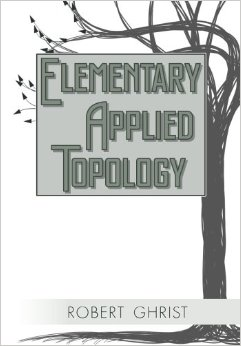This book seems like it is from 10 years in the future. A glimpse of things to come?

A quote from this interview with Ghrist,

Homology is kind of old school…

Counting clusters and holes (homology) in data is useful, but not groundbreaking mathematics, and is not the extent of what topology can do. If one regards current TDA results as unconvincing, this should not be taken as sufficient evidence against the utility of topological thinking in applied sciences.

To get a sense of promising new directions, consider having a system of equations parameterized by an underlying topological space. Such objects are ubiquitious when the underlying topological space is a graph: probabilistic graphical models, computational graphs, etc. How do the solutions to these equations depend on the underlying topological features of the space? This leads to sheaf theory, which has seen a recent surge of publications in the applied topology literature. Introductions to applied sheaf theory include,

Sheaf theory extends our tools from TDA (e.g. homology) by attaching algebraic objects to the open sets of the space. Instead of just a simplicial complex, we have, say, vector spaces over the simplicial complex, together with functions to move us around the topological space. Augmenting the “mere” topological spaces of TDA with algebraic structure seems sensible enough. And, at minimum, sheaf theory would give us a language to define local versus global solutions to the system of equations, obstructions in extending local solutions to global solutions, and how this all depends on the topology of the underlying space.

## Resources

There are a few go-to references for TDA, including,

My personal favorite is a recently published IEEE magazine article,

which I highly recommend if you are to only read one.

For applied topology more generally, I recommend the excellent preprint,

## Textbooks

Of the available TDA-relevant textbooks, I prefer,

## Software

Recommended software includes,

## Discussion

In the classical period, people on the whole would have studied things on a small scale, in local coordinates and so on. In [the 20th] century, the emphasis has shifted to try and understand the global, large-scale behaviour. And because global behaviour is more difficult to understand, much of it is done qualitatively, and topological ideas become very important. It was Poincare who both made the pioneering steps in topology and who forecast that topology would be an important ingredient in 20th-century mathematics. Incidentally, Hilbert, who made his famous list of problems, did not. Topology hardly figured in his list of problems. But for Poincare it was quite clear it would be an important factor – Sir Michael Atiyah

Topology is uniquely suited for defining and computing the global/nonlocal properties of spaces. 20th century (pure) mathematics had a need for this. It will be interesting to see if 21st century applied mathematics will see a similar need for the passage from local to global. If so, then TDA / applied topology will continue to grow as a research field. Out of necessity, not fashion.